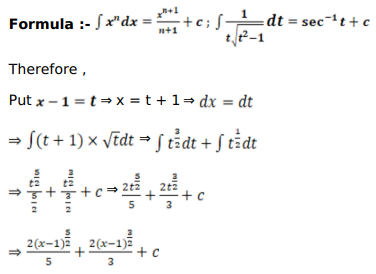# Mark against the correct answer in each of the following:

Question:

Mark $(\sqrt{)}$ against the correct answer in each of the following:

$\int x \sqrt{x-1} d x=?$

A. $\frac{2}{3}(x-1)^{3 / 2}+C$

B. $\frac{2}{5}(x-1)^{5 / 2}+C$

C. $\frac{2}{5}(x-1)^{5 / 2}+\frac{3}{2}(x-1)^{3 / 2}+C$

D. none of these

Solution: